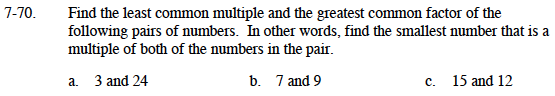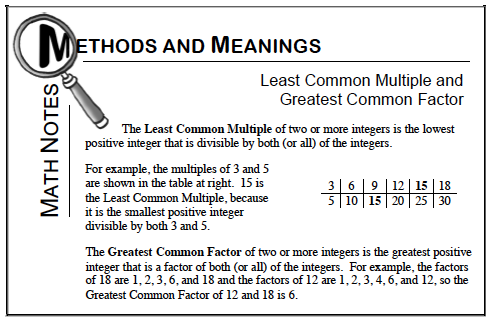### Home > MC1 > Chapter 7 > Lesson 7.2.3 > Problem7-70

7-70.Use the Math Notes box below to help you with these problems.

LCM is 24. GCF is 3.

Again, refer to the Math Notes box.

7 is a prime number, which means its only factors are 1 and itself.

Refer to the Math Notes box.

LCM is 60. Can you find the greatest common factor?